Courses

Test: Number - 2

20 Questions MCQ Test Mathematics for Class 1: NCERT | Test: Number - 2

Description
Attempt Test: Number - 2 | 20 questions in 40 minutes | Mock test for Class 1 preparation | Free important questions MCQ to study Mathematics for Class 1: NCERT for Class 1 Exam | Download free PDF with solutions
QUESTION: 1

Solution:
QUESTION: 2

Solution:
QUESTION: 3

Identify the wrong option from the following subtractions.

Solution:
QUESTION: 4

Complete the series. 24, 23, 22, 21, ?

Solution:
QUESTION: 5

What is 9 tens and 6 ones?

Solution: 96
QUESTION: 6

Identify the odd one out.

Solution:
QUESTION: 7

Complete the following series from the options given bvelow.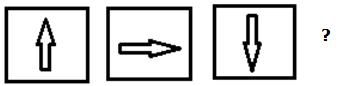Solution:
QUESTION: 8

Which number comes in between 39 and 41?

Solution:
QUESTION: 9

Which of the following shape is different from the other optins?

Solution:
QUESTION: 10

Complete the number series.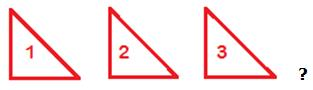Solution:
QUESTION: 11

A basket has space for keeping 10 eggs but only 4 eggs are kept in it. How many more eggs you can put in it?

Solution:

6 more eggs we can put in it.

QUESTION: 12

Identify the odd one out.

Solution:
QUESTION: 13

Complete the following serise.

Rs.100, Rs.200, Rs.300, Rs.400, Rs.500, Rs.?

Solution:
QUESTION: 14

Identify the missing number in the following series.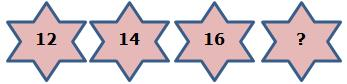Solution:
QUESTION: 15

Which of the following shape is different from the other opitions.

Solution:
QUESTION: 16

Complete the given series from the options given below.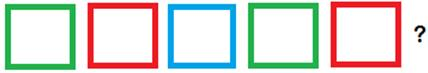Solution:
QUESTION: 17

Which is the smallest number in the follwoing options?

Solution:

A. 20, B. 25, C. 27,
A is correct.

QUESTION: 18

Which of the following series is not in order?

Solution:
QUESTION: 19

Complete the given series from the following options.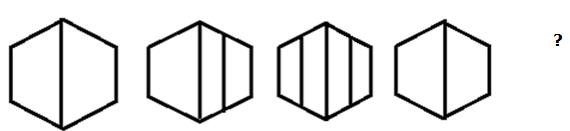Solution:
QUESTION: 20

Identify the missing number in the following ascending number order.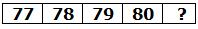Solution:Use Code STAYHOME200 and get INR 200 additional OFF Use Coupon Code

Track your progress, build streaks, highlight & save important lessons and more!

Similar ContentRelated tests